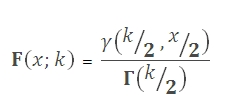# SQL Server CHISQ.DIST function

Updated: 9 August 2010

Use CHIDIST to calculate the one-tailed probability of the chi-square distribution. The chi-square distribution is associated with a chi-squared result. The chi-square distribution is a special case of the gamma distribution. Its cumulative distribution function is:Syntax
SELECT [wctStatistics].[wct].[CHIDIST] (
<@X, float,>
,<@Degrees_freedom, float,>)
Arguments
@X
is any real positive number. @X is an expression of type float or of a type that can be implicitly converted to float
@Degrees_freedom
is any real positive number. @Degrees_freedom is an expression of type float or of a type that can be implicitly converted to float.

Return Types
float
Remarks
·         If @X < 0, CHIDIST returns an error.
·         @Degrees_freedom is truncated to zero decimal places
·         if @Degrees_freedom < 1 or @Degrees_freedom > 10^10, CHIDIST returns an error.
Examples

select
wct.CHIDIST(15.0862724691398, 5)

This produces the following result

----------------------
0.0100000000000011

(1 row(s) affected)

This produces the following result

----------------------
0.0100000000000011

(1 row(s) affected)

### SupportCopyright 2008-2023 Westclintech LLC         Privacy Policy        Terms of Service